# How to use MAX CASE WHEN in SQL

## Overview

Currently, as of MySql 8, there are more than 700 reserved keywords, each with its unique function. While memorizing them is impossible, knowing essential ones, such as the `MAX CASE WHEN` keyword combo, is essential.

`CASE WHEN` allow data scientists to create a pseudo if…else statement in SQL. These control structures direct data flow and modify how data and instructions work through these boolean-based structures.

Below, we introduce the concept of `MAX CASE WHEN` for answering SQL Questions.

## MIN and MAX Functions

Before we head into the `MAX CASE WHEN` discussion, let us first understand the underlying concept within the MAX function.

At its core, the `MAX` function goes through a column and gets its maximum value.

When given column A represented by a set x where x = {3, 21, 412, 21, -1234, 133}, inputting column A inside `MAX` will return 412. The same can also be said with the `MIN` function. Given the same set x, `MIN(x)` will return -1234.

## SQL Control Structures: CASE WHEN

SQL control structures are ways to control the program flow, allowing SQL to function like a structured programming language.

Before we proceed with the different uses of nested control structures like `MAX(CASE WHEN)`, `MIN(CASE WHEN)`, and `CASE WHEN MAX()`, we should familiarize ourselves with the `CASE WHEN` syntax.

### Case when… then end

The `CASE` statement initializes the control structure, telling the SQL interpreter that a series of `WHEN` statements are to follow. `WHEN` specifies a series of conditions that can be evaluated as either true or false.

When the condition inside the `WHEN` statement is met (i.e., true), the `THEN` statement is then executed. Unlike most programming languages, when a `CASE` statement is verified as accurate, it automatically stops and does not look for a break directive.

``````CASE WHEN <STATEMENT IS TRUE> THEN 'THIS IS EXECUTED'
WHEN <THIS STATEMENT ALSO RETURNS TRUE> THEN 'THIS IS NOT EXECUTED'
``````

The `ELSE` keyword in SQL handles the control whenever all the `CASE` statements fail, i.e., when all the `CASE` statements do not manage to return true. It plays an integral role in handling edge cases and exceptions.

To consider edge cases, we use the `ELSE` keyword. Given the following code:

``````Select inflated_price,
Case when inflated_price < 35 then 'The price is okay.'
when inflated_price < 50 then 'It is starting to sting'
when inflated_price < 70 then 'Expensive'
ELSE 'Very Expensive!' END AS Remarks
from Products;
``````

We can see that the output is: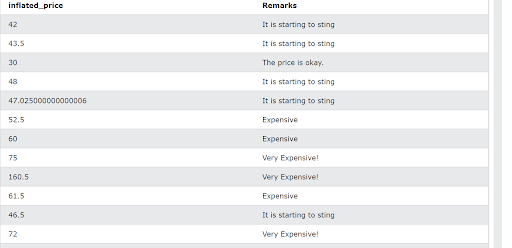As you can see, `NULLs` are removed because the `ELSE` statement handles all the edge cases. `ELSE` is excellent at handling data beyond the bounds of your current control structure’s scope and creating a default output for them.

If there were no `ELSE` statement, all prices equal to or above 70 would return a result of `NULL`.

`MAX CASE WHEN` is a sequence of commands and functions whose usefulness may only be apparent at a glance. However, many use cases for `MAX CASE WHEN` are incredibly useful, albeit pretty niche.

### Case 1: Finding the maximum value under a threshold

We can use `MAX(CASE WHEN)` to find the maximum value in a table under a threshold. Supposing you want to find the most expensive priced item below 60, given the previous table, we can do the following query:

``````Select inflated_price,
MAX(Case when inflated_price < 60 then inflated_price end)
from Products;
``````

We get the following result: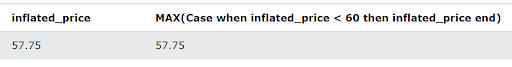As you can see, instead of returning the `MAX` value of the table, it returns only the maximum value below the 60 threshold. This approach can be great for finding values within a specific range, and we can also do the inverse with the min function.

### Case 2: Finding the minimum value under a threshold

Let us formulate a hypothetical scenario: Your organization wants to find the cheapest, decently-priced quality item, and the said organization has decided that any item priced below 80 is too cheap, as it may severely reflect on the product quality (quality isn’t necessarily correlated with price, but stick with us for this particular scenario).

You then run the following query:

``````Select inflated_price,
MIN(Case when inflated_price > 80 then inflated_price end) as idealprice
from Products;
``````

We get the following result: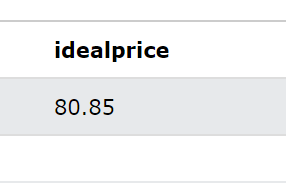### Case 3: Date Handling with MAX

The `MAX` function is versatile and can return more than just numeric values; it can also work with unique identifiers, characters, and dates. We will be learning about `MAX(CASE WHEN)` with dates for this case.

When taking a date as a parameter, the `MAX` function returns the latest data available, while the `MIN` function returns the converse. Let us look at the following scenario as an example:

Company ABC decided to trace their orders placed within the year 1996 and would like to find the last order that year as a specific anomaly happened with that particular order. To find the previous order on that day, you can use the following query:

``````Select MAX(Case when OrderDate < '1996-12-31' then OrderDate end) as latest_possible_order from Orders
``````

This will result in the following: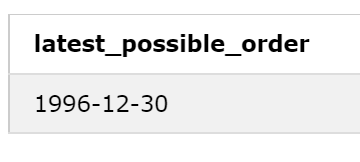Conversely, we can also find the earliest order date of a particular year by modifying our query accordingly.

``````Select MIN(Case when OrderDate > '1996-12-31' then OrderDate end) as earliest_possible_order from Orders
``````

Which results in the following: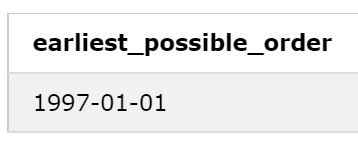### Case 4: CASE WHEN MAX(), conditionals on the max value

While we touched on the use cases of `MAX(CASE WHEN)`, let us explore the possible uses for its inverse (i.e., `CASE WHEN MAX())`. Given the following situation:

You have a list of records of the number of points a customer has accumulated per month over the last five years. You would want to determine whether or not a customer is a VIP customer when their `MAX` amount of points during one month exceeds 60.

Moreover, we also add conditionals determining if they are a GOLD customer (`MAX points > 40`), a silver customer (`MAX points > 20`), or just a regular customer.

We run the following query:

``````select points,
CASE WHEN MAX(points) > 60 then 'VIP CUSTOMER'
WHEN MAX(points) > 40 then 'Gold CUSTOMER'
WHEN MAX(points) > 20 then 'Silver CUSTOMER'
ELSE 'REGULAR CUSTOMER'
END as customer_royalty
from customer_points;
``````

We get the following result: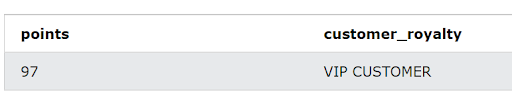Because the customer has accumulated a maximum of 97 points within a one-month period, they are considered a VIP CUSTOMER.

Aside from the usuals, we also have more examples wherein we can utilize the `CASE WHEN MAX` combo. Given the following list:

Creating your sorting algorithm using SQL. While using `ORDER BY` is recommended, when you want to mutate a column’s value while not the other values related to the said column, `MAX()` and `MIN()` can help you with that.

It is also helpful when you want to implement your sorting technique with relative ease (i.e., alternating `MAX()` and `MIN()` together to create an alternating-sort column). `MAX (CASE WHEN CASE WHEN)`, i.e., nested `CASE WHENs`. When you want another value to decide the value of another value, you use nested `CASE WHENs`. For example:

``````		CASE WHEN 'X is true'
THEN CASE
WHEN 'A is true' THEN <analyze this row>
WHEN 'B is true' THEN <analyze this row>
ELSE CASE
WHEN 'C is true" THEN <analyze this row>
``````

## Training for SQL

There’s always a saying among data scientists and data analysts that getting started with SQL is relatively easy, but mastering the language is a lifelong process.

One of the best ways to learn SQL is to write your queries by solving hypothetical problems. You can view SQL interview questions on Interview Query’s website.

Here are a few examples:

### 1. Duplicate Rows

Given a users table, write a query to return only its duplicate rows.

Input:

`users` table

Column Type
`id` INTEGER
`name` VARCHAR
`created_at` DATETIME

Code solution:

``````SELECT
id,
name,
created_at
FROM (
SELECT
*,
row_number() OVER
(PARTITION BY id ORDER BY created_at ASC)
AS ranking
FROM
users) AS u
WHERE
ranking > 1
``````

### 2. Student Tests

Let’s say there are two tables, students and tests.

The tests table does not have a student id. However, it has both the first and last name and date of birth, which we consider inaccurate since these details are entered manually.

What process would you use to determine which student in the student rosters took which exam?

Note: You can assume that you can utilize a human to support the review of your matches and that you need to evaluate thousands of rows of data for this project.

Input:

`students` table

Columns Type
`id` INTEGER
`firstname` VARCHAR
`lastname` VARCHAR
`date_of_birth` DATETIME

Output:

`tests` table

Columns Type
`firstname` VARCHAR
`lastname` VARCHAR
`date_of_birth` DATETIME
`test_score` INTEGER
`test_date` DATETIME

Most popular answer from Interview Query:

I would start with the assumption that making a mistake on more than one column is unlikely. I would select a candidates table where each test has several rows, each consisting of students with at least two matching columns. To choose from the candidates, I would use a dictionary of common typographical mistakes for the names and dates. If all three match, I would select that option. Otherwise, go with a measure of the difference between the values.

If two identically named students are transposed, then the only recourse would be manual selection.

Another user-submitted answer showcased the following:

Other than exact matches for `firstname` + `lastname` + `dateofbirth`, I think you’d want to look for matches to 23 and have a human review the third field to see if it approximately matches.

For example, for Jon Doe, born on 5/7/1990, you might match Jonathan Doe on 5/7/1990 or Jon Doe on 6/7/1990.

I’d expect an exact match on 33 fields to net ~80% of matches, then need 23 matching to get another ~15%.

Finally, if it’s only thousands of rows, you should be able to hand-match the remaining ~5%. I’d sort by the last name since it’s more likely to be unique than the first name and is less susceptible to a fat-finger mistake than DOB. (Even if you slightly misspell the last name, it will look similar enough that a human can spot a match.)

Using the second solution, let us build a pseudo code SQL query to accomplish precisely that.

Concatenate the `firstname`, `second name`, and `date_of_birth` columns from the `Students_table`, and store this concatenation into a new column. For ease of reference, we can call this column ‘compare_A’. By aggregating our relevant data, we can easily compare each student’s information.

Do the same, but this time from the `tests_table`: Concatenate the first name, second name, and `date_of_birth` columns. For ease of reference, we can call this concatenated column `compare_B` Fuzzy match an element from `compare_A` to all the elements of `compare_B` using the Levenshtein Distance algorithm.

To get all the values that match 66% (23) of our original string, get the length of the longer string (i.e. if an element from `compare_B` is more extended, use that element’s length). Using the length, divide the Levenshtein distance’s result by the length. If a resulting calculation for two elements is greater than 33% (i.e., 13), exclude them from the list. Use manual review to match the two tables’ results from each other.